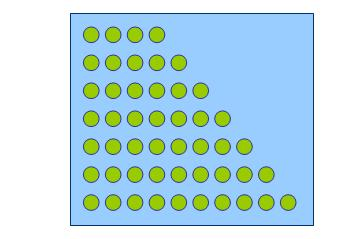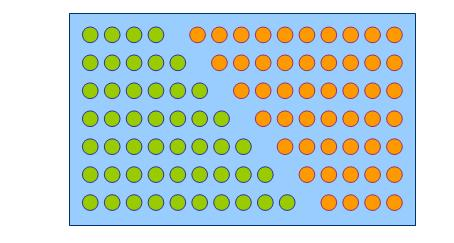#### You may also like### Diophantine N-tuples

Can you explain why a sequence of operations always gives you perfect squares?### DOTS Division

Take any pair of two digit numbers x=ab and y=cd where, without loss of generality, ab > cd . Form two 4 digit numbers r=abcd and s=cdab and calculate: {r^2 - s^2} /{x^2 - y^2}.### Sixational

The nth term of a sequence is given by the formula n^3 + 11n . Find the first four terms of the sequence given by this formula and the first term of the sequence which is bigger than one million. Prove that all terms of the sequence are divisible by 6.

# Reasonable Algebra

##### Age 14 to 16 Challenge Level:

This problem builds on Consecutive Sums and invites you to explore how algebra can help with proof. If you are not familiar with the problem it would be good to look at that first.

12 can be made as a sum of consecutive numbers: 3 + 4 + 5.

15 can also be made as a sum of consecutive numbers in several ways, for example 7 + 8.

At first it seems that maybe any total could be made as a sum of consecutive numbers. However, after trying several possibilities, it doesn't seem as though we can make 16 in this way.

Every total from 17 to 31 can be made as a sum of consecutive numbers, until we reach 32.Let's use algebra to explore why that is.

First : what is the sum of 7 consecutive numbers starting with 4?

There is a quick way to calculate consecutive sums. Do you know it?

Take a look at the next image.Take the set to be counted twice, the second time inverted.

So the sum here $4+5+6+7+8+9+10= \frac{1}{2}\times (4+10) \times 7$

Can you use this method to find the sum of 8 consecutive numbers starting at 10 ?

Here's a much longer run of consecutive numbers, can you find its sum : 10, 11, 12, . . . . . . . . . 79, 80?

What is the sum of n consecutive numbers starting at s ?

This might help you to use algebra to reason why 16 and 32 are impossible to create as the sum of consecutive numbers.

Can you make a general statement about numbers of that sort (powers of 2)?

##### Note:
Why 24 ? is another problem where reasoning from algebra proves to be very powerful.